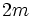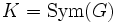# Order is twice an odd number implies subgroup of index two

## Statement

Suppose$G$ is a finite group and the order of$G$ is$2m$, where$m$ is an odd number. Then,$G$ has a Subgroup of index two (?), i.e., a subgroup of order$m$.

Note that since index two implies normal, the subgroup of order$m$ is in fact a normal subgroup, and is thus the Brauer core (?) of the whole group.

## Related facts

### Stronger facts

For a complete list of normal p-complement theorems, refer:

### Related facts about index two and least prime index

• Index two implies normal
• Subgroup of least prime index is normal: If$p$ is the least prime dividing the order of a finite group$G$, any subgroup of index$p$ is normal.
• Normal of least prime order implies central

## Facts used

1. Cayley's theorem: Any group$G$ can be embedded inside the symmetric group$\operatorname{Sym}(G)$, where an element$g \in G$ acts by left multiplication.
2. Cauchy's theorem: If a prime$p$ divides the order of a finite group$G$, then$G$ has an element of order$p$.
3. Index satisfies transfer inequality: If$A,B$ are subgroups of$C$, then$[A:A \cap B] \le [C:B]$.

## Proof

### Elementary proof

Given: A finite group$G$ of order$2m$, where$m$ is an odd integer.

To prove:$G$ has a subgroup of index two.

Proof: By fact (1), consider the embedding of$G$ as a subgroup of$K = \operatorname{Sym}(G)$. Let$L = \operatorname{Alt}(G)$ be the alternating group on$G$. By definition$L$ is a subgroup of index two in$G$.

1.$G$ contains an element, say$g$, of order two: This follows from fact (2).
2.$g$, viewed as an element of$K = \operatorname{Sym}(G)$, is an odd permutation. In other words,$g \notin L$: The cycle decomposition consists of$m$ cycles of length two each, i.e., an odd number of cycles of even length. Thus,$g$ is an odd permutation.
3.$G \cap L$ is a subgroup of index two in$G$: By fact (3),$G \cap L$ has index either one or two in$G$. However, the previous step shows that$G$ is not contained in$L$, so$G \cap L$ is a proper subgroup of$G$. Thus,$G \cap L$ is a subgroup of index two in$G$.